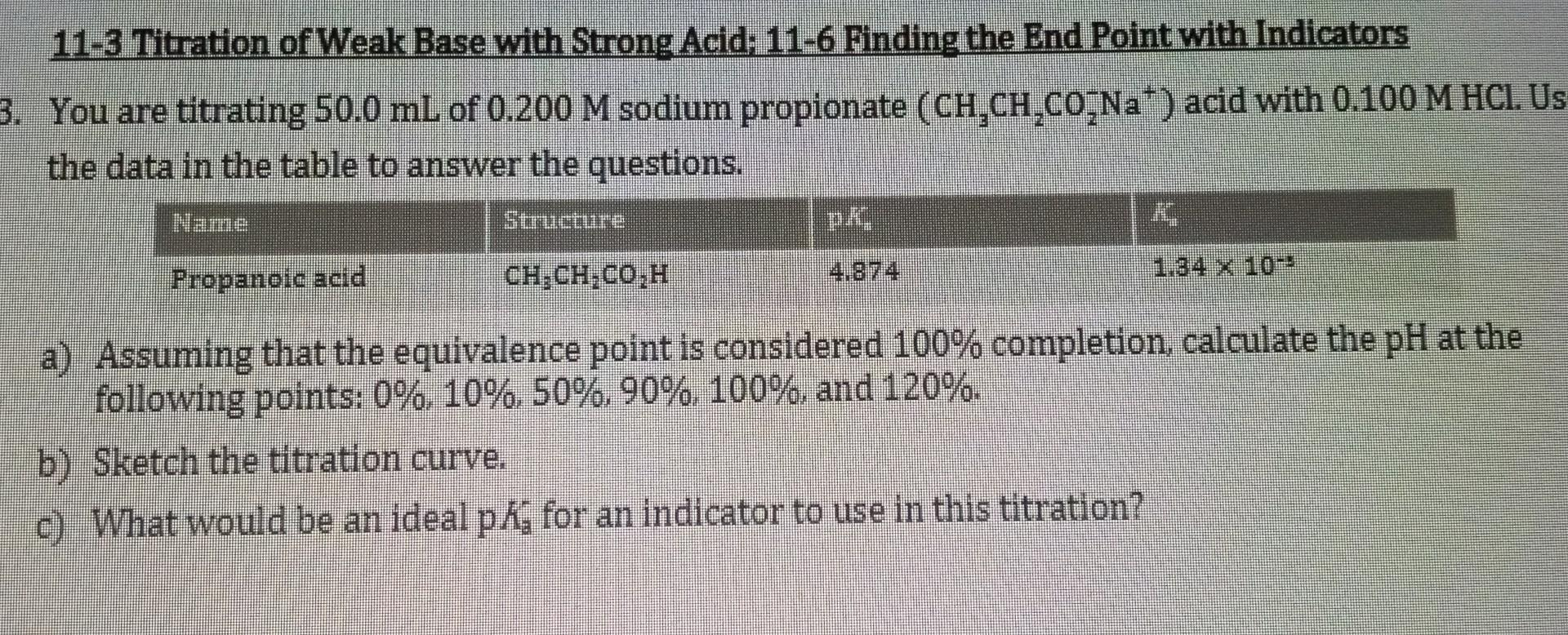# Question   11-3 Titration of Weak Base with Strong Acid; 11-6 Finding the End Point with Indicators You are titrating $$50.0 \mathrm{~mL}$$ of $$0.200 \mathrm{M}$$ sodium propionate $$\left(\mathrm{CH}_{3} \mathrm{CH}_{2} \mathrm{CO}_{2}^{-} \mathrm{Na}^{+}\right)$$acid with $$0.100 \mathrm{M} \mathrm{HCl}$$. Us the data in the table to answer the questions. \begin{tabular}{|l|l|l|l|} \hline Name & Structure & $$\mathrm{pK}$$ & $$\mathrm{K}$$ \\ \hline Propanoic acid & $$\mathrm{CH}_{3} \mathrm{CH}_{2} \mathrm{CO}_{2} \mathrm{H}$$ & 4.874 & $$1.34 \times 10^{-3}$$ \\ \hline \end{tabular} a) Assuming that the equivalence point is considered $$100 \%$$ completion, calculate the $$\mathrm{pH}$$ at the following points: $$0 \%, 10 \%, 50 \%, 90 \%, 100 \%$$, and $$120 \%$$. b) Sketch the titration curve.Transcribed Image Text: 11-3 Titration of Weak Base with Strong Acid; 11-6 Finding the End Point with Indicators You are titrating $$50.0 \mathrm{~mL}$$ of $$0.200 \mathrm{M}$$ sodium propionate $$\left(\mathrm{CH}_{3} \mathrm{CH}_{2} \mathrm{CO}_{2}^{-} \mathrm{Na}^{+}\right)$$acid with $$0.100 \mathrm{M} \mathrm{HCl}$$. Us the data in the table to answer the questions. \begin{tabular}{|l|l|l|l|} \hline Name & Structure & $$\mathrm{pK}$$ & $$\mathrm{K}$$ \\ \hline Propanoic acid & $$\mathrm{CH}_{3} \mathrm{CH}_{2} \mathrm{CO}_{2} \mathrm{H}$$ & 4.874 & $$1.34 \times 10^{-3}$$ \\ \hline \end{tabular} a) Assuming that the equivalence point is considered $$100 \%$$ completion, calculate the $$\mathrm{pH}$$ at the following points: $$0 \%, 10 \%, 50 \%, 90 \%, 100 \%$$, and $$120 \%$$. b) Sketch the titration curve.
Transcribed Image Text: 11-3 Titration of Weak Base with Strong Acid; 11-6 Finding the End Point with Indicators You are titrating $$50.0 \mathrm{~mL}$$ of $$0.200 \mathrm{M}$$ sodium propionate $$\left(\mathrm{CH}_{3} \mathrm{CH}_{2} \mathrm{CO}_{2}^{-} \mathrm{Na}^{+}\right)$$acid with $$0.100 \mathrm{M} \mathrm{HCl}$$. Us the data in the table to answer the questions. \begin{tabular}{|l|l|l|l|} \hline Name & Structure & $$\mathrm{pK}$$ & $$\mathrm{K}$$ \\ \hline Propanoic acid & $$\mathrm{CH}_{3} \mathrm{CH}_{2} \mathrm{CO}_{2} \mathrm{H}$$ & 4.874 & $$1.34 \times 10^{-3}$$ \\ \hline \end{tabular} a) Assuming that the equivalence point is considered $$100 \%$$ completion, calculate the $$\mathrm{pH}$$ at the following points: $$0 \%, 10 \%, 50 \%, 90 \%, 100 \%$$, and $$120 \%$$. b) Sketch the titration curve.